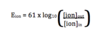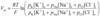M&R S3 - The Resting Membrane Potential Flashcards Preview

► Med Misc 01 > M&R S3 - The Resting Membrane Potential > Flashcards

Flashcards in M&R S3 - The Resting Membrane Potential Deck (20):
1

2

3

4

5

6

7

8

9

What is the Nernst equation? Hint: Assume 37 degrees celsius

Ions in = concentration of ions inside the cell Ions out = Concentration of ions outside the cell10

11

12

13

14

Explain what is calculated by the Goldman-Hodgkin-Katz equation Write out the equation, explain the meaning of any symbols present

The GHK equation provides a good approximation of membrane potential as determined by Na+, K+ and Cl- ions Vm = Membrane potential PNa PK  PCl  = Relative permeabilities of the ions [ion] = concentration of the ion R = Gas constant T = Temperature (celsius) F = Faraday's number15

16

17

18

19

20